# FUNCTIONS IN PYTHON PROGRAMMING

in STEMGeeks2 months ago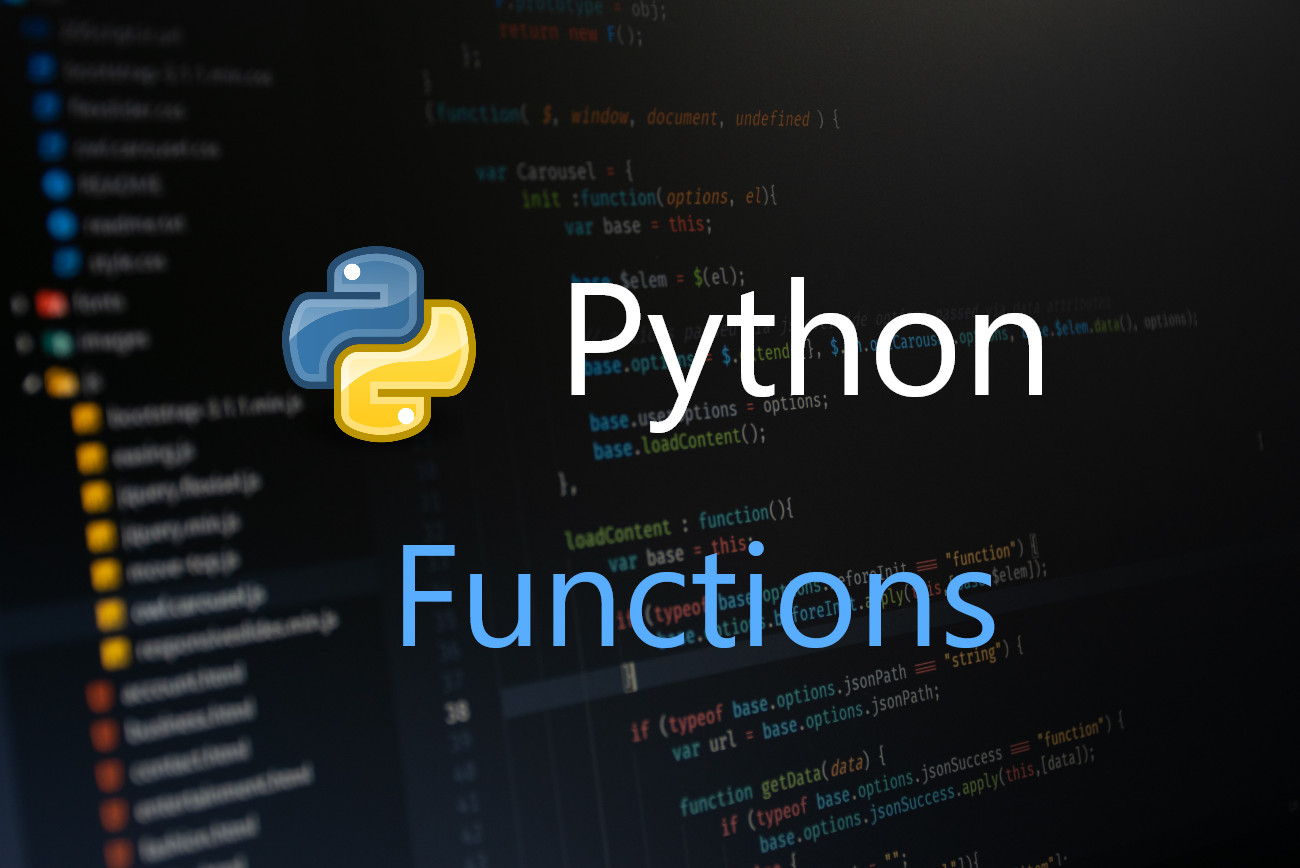Image Source

In every programming language, function is an importance concept. It is basically a chunk of organized code that is called in order to perform some certain actions. In other words, we use functions so as not to repeat the certain block of codes again and again. It provides the advantage of code reusability to the programmer and makes the code clear, concise, and modular. Your function can be called from any other parts of your program. In order to use this concept, you need to define your function specifying the task it does and in order to invoke it into your program, you need to call it. Almost in every programming language, functions take parameter inside round brackets "()". Functions utilize these parameters value to do the task and while calling, we need to pass arguments too so that function can obtain the output we have defined.

Understanding function is pretty much easier in Python than any other programming languages. It is defined using `def` keyword and then after that we write the name of function and put a colon after the function name. Below is a simple example of function.

``````def simple_function():
print("Hello!")

simple_function()
``````

In this code, our function name is `simple_function`. It need no parameter to do its task. So we have used an empty parenthesis. The line after your function definition is called Function Body. The function body contains the actual task to be executed when the function is called and in our case is to print Hello!. And at last, we have called our function name.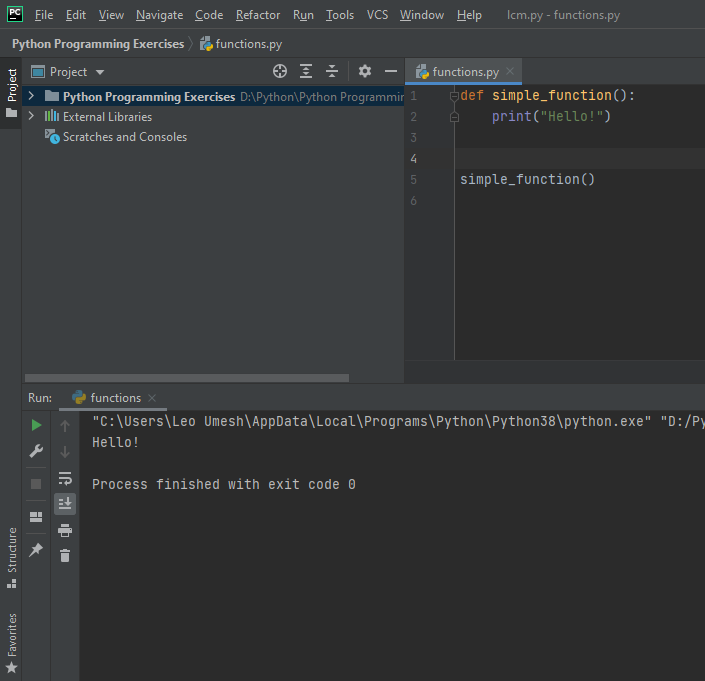Now lets modify this function slightly to understand what function parameters and arguments are.

``````def simple_function(name):
print("Hello, " + name)

simple_function("Jack")
``````

The variable `name` in the above function definition is called parameter i.e. a value that is needed for a function to do its actual task. And the value `Jack` is an argument and it gets passed from the function call to the actual function. And the name in the function definition gets replaced with Jack.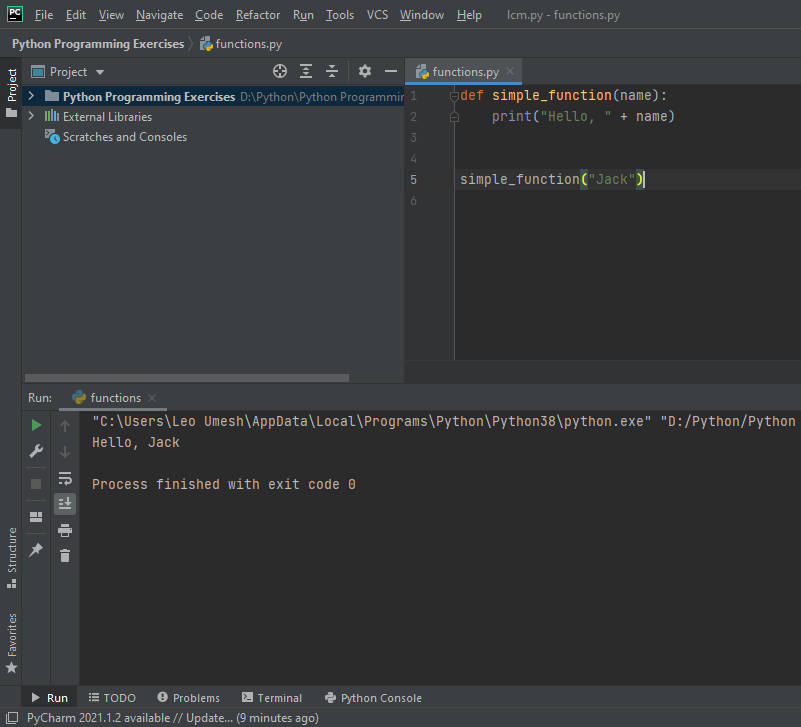You can use any number of parameter you like depending on the programs you are building. If you don't know how many to specify in the function parameters, python has provided a special feature to help with this one too. I will be discussing this later on another post as it is a lengthy post.

Lets see another simple example of function that contains if-else syntax. We will divide two number and if the divisor is 0 then, we want to specify that the number can't be divided by 0 as it is not mathematically possible. If divisor is other than 0, then we want to do the division. Here's the code:

``````def divide(x, y):
if y == 0:
print("Can't divide a number by zero.")
else:
z = x / y
print("The division result is: ", z)

a = int(input("Enter a dividend: "))
b = int(input("Enter a divisor: "))

divide(a, b)
``````

Lets see by providing values other than 0 for both dividend and divisor.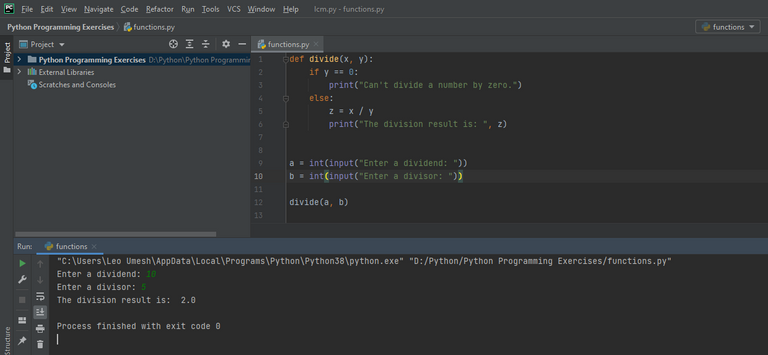If you enter 0 for divisor, then you will get the following message on the output screen.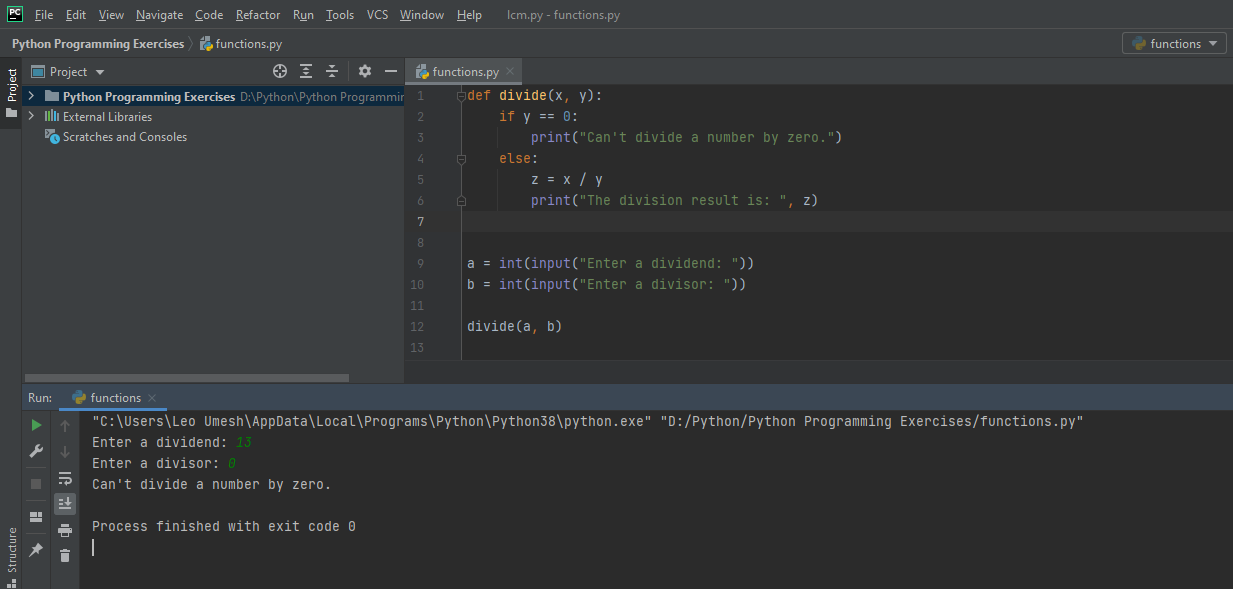The next thing I will like to talk about function is the types of argument. There are three types of argument in python functions. They are:

• Positional Arguments
• Keyword Arguments
• Default Values

### 1. Positional Arguments

Consider the following code which prints the person name and address.

``````def info(name, address):
print("Hey, " + name + "." + "You live in " + address)

info("Jack", "Delhi")
``````

In this code, the argument "Jack" is assigned to the parameter "name" and "Delhi" is assigned to the parameter "address". Argument values are stored in the order as defined in the parameter. Providing arguments in this way is called Positional Argument. The output can be seen as below: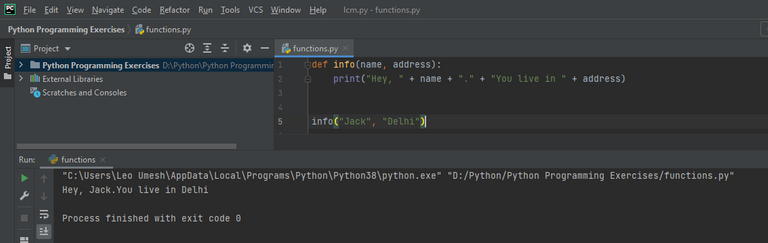### 2. Keyword Arguments

If you want to pass a value to a function by specifying its parameter name in the function call, then it is called Keyword Arguments. It is a name-value pair that is passed during a function call. Lets see the example below to calculate the area of a circle.

``````def circle_area(radius, pi):
print("The area of a circle is: ", a)

``````

Here, we are telling the python that we want to assign 3.14159 to the parameter named as "pi" and value 5 to the parameter named as "radius". If you explicitly defined the values you want to assign to the specific parameter, then it is called Keyword Argument. See the output below: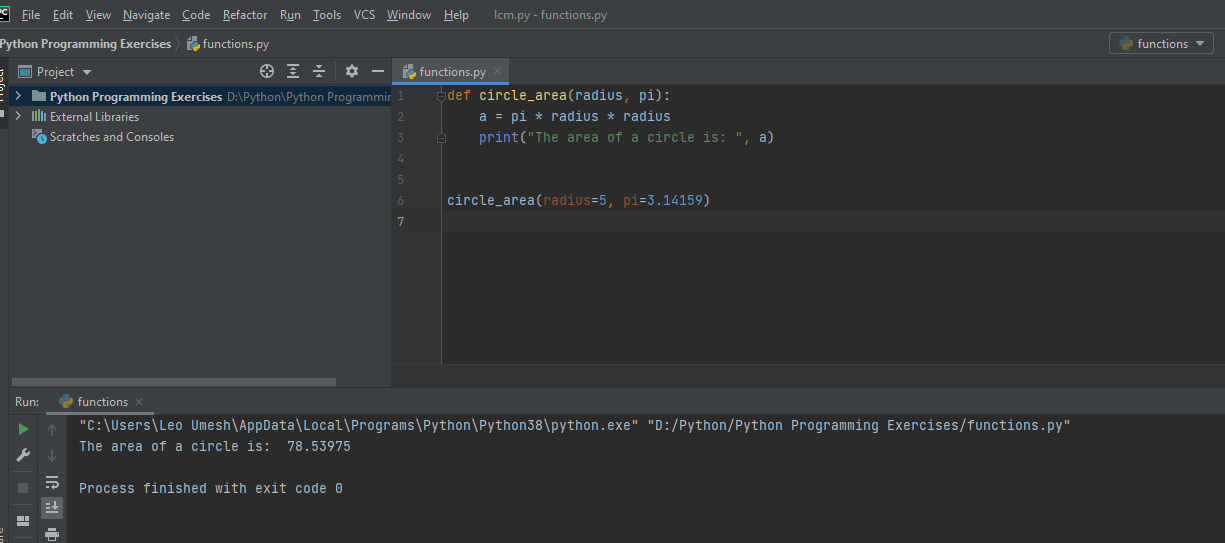Also note here that the order doesn't matter. You can call the function with this syntax too: `circle_area(pi=3.14159, radius=5)`. The output is same as the above: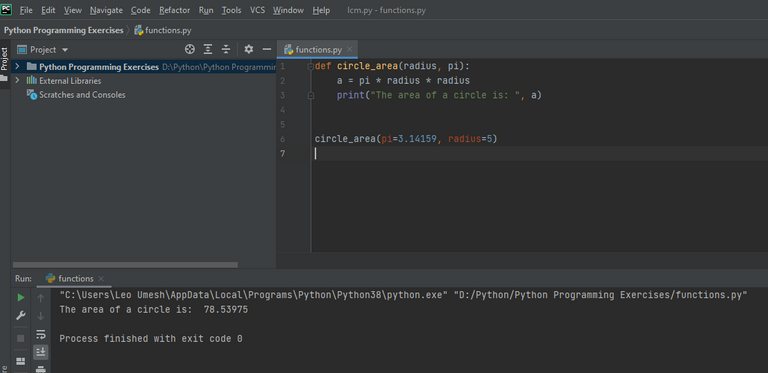### 3. Default Values

In the above code for finding the area of a circle, the value of pi is always constant. We don't need to specify its value again and again as an argument during a function call. Rather we can tell that the default value of pi is 3.14159 and user doesn't need to pass the argument value of pi during the function call. This way of passing an argument is called "Default Value". See the code below for example:

``````def circle_area(radius, pi=3.14159):
print("The area of a circle is: ", a)

circle_area(5)
``````

Here we already specify the default value for pi in the function definition. So you don't need to pass pi value in the argument and you can see we only passed the radius as 5. Hope you grasp the concept.

The output when the program is executed is as below: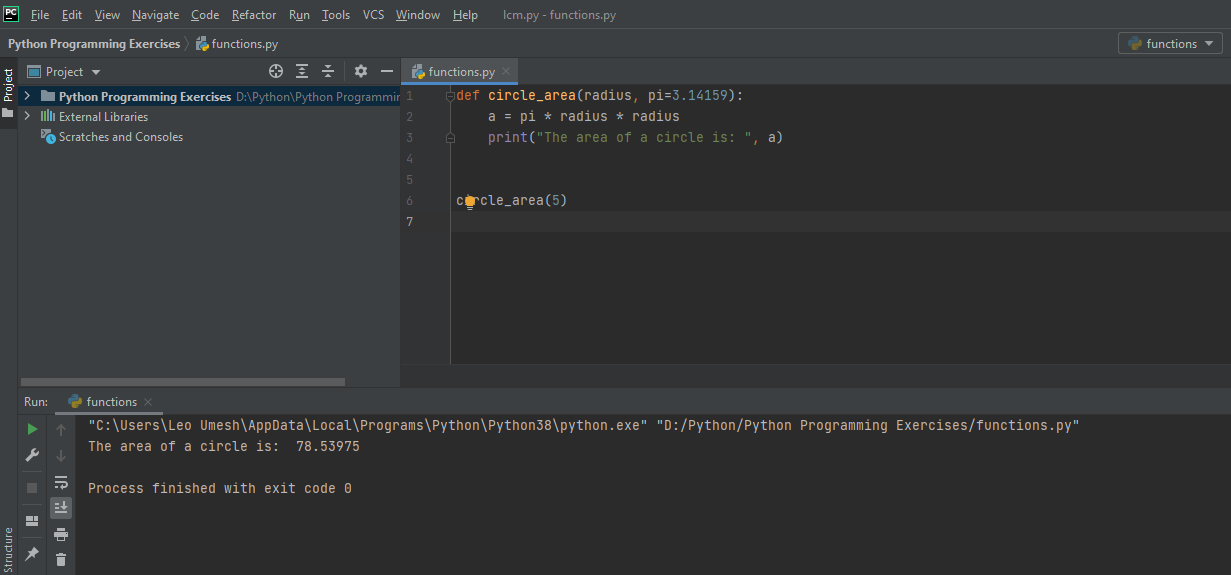So, I would like to end this post here as of now. If you have any doubt or confusion, please let me know in the comment. I would be glad to help you. Cheers!

Sort:

Your content has been voted as a part of Encouragement program. Keep up the good work!

Use Ecency daily to boost your growth on platform!

Support Ecency
Vote for Proposal
Delegate HP and earn more

Thank you🙂